# 二次方程画图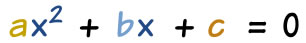abc 可以是任何值，除了 a 不能是 0。）## 最简单的二次式

f(x) = x2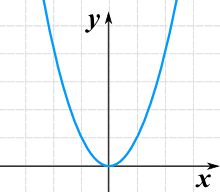f(x) = ax2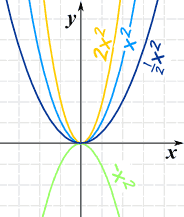• 大的 a 值把曲线向内压缩
• 小的 a 值把曲线向外扩大
• 负数的 a 把曲线上下倒转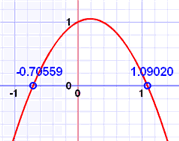## 来玩玩

"探索二次方程" 这页玩玩。在那里，你可以

## "一般的"二次式

f(x) = ax2 + bx + c

f(x) = a(x-h)2 + k

• h = -b/2a
• k = f( h )

## 对不起，请不要介意我问……做这个来干什么？

 把方程写成这样的妙处是： h 和 k 告诉我们曲线的最低（或最高）点，叫 顶点： 同时，曲线是沿 x=h 轴 对称 的，这使绘图也比较容易### 如下……

• h 告诉我们曲线从 x=0 向左（或右）移了多远
• k 告诉我们曲线从 y=0 向上（或下）移了多远

### 例子：画 f(x) = 2x2 - 12x + 16 的图

• a = 2,
• b = -12,
• c = 16

• a 是正数，所以曲线"向上" （像英语字母"U"）
• a 是 2，所以图是比 x2 的图"压缩"了一点

h = -b/2a = -(-12)/(2x2) = 3

k = f(3) = 2(3)2 - 12·3 + 16 = 18-36+16 = -2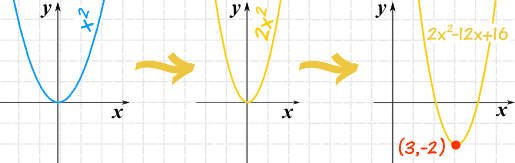## 从图到方程

### 例子：你用一些数据画了个图，而图看上去像个二次式：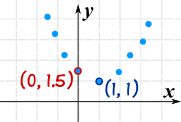(h, k) = (1,1)

f(x) = a(x-h)2 + k

f(x) = a(x-1)2 + 1

 我们知道线上有一点是 (0, 1.5)，所以： f(0) = 1.5 除了 a 以外，我们也知道函数： f(0) = a(0-1)2 + 1 = 1.5 简化： f(0) = a + 1 = 1.5 a = 0.5

f(x) = 0.5(x-1)2 + 1